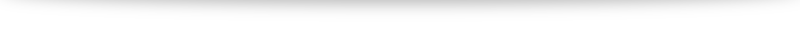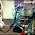# Strategy Design Pattern

Design patterns are reusable solutions to repetitive problems. On various occasions I have seen people writing conditional code. Design patterns help in modularizing the code and making it extensible and maintainable. This post is about how we can refactor conditional logic to better maintainable and cleaner solution using Strategy Design Pattern.

### Problem statement

Imagine we have to calculate the monthly instalment for a loan. The loan can be of various types for e.g. home loan, car loan, personal loan etc. Each loan can have different duration and also different interest rates. Based on these factors the equated monthly instalments (EMI) will be different. Lets look at a solution without using design patterns and try and refactor the same to one using design patterns.

For this hypothetical example lets consider that the interest rates for home loan is 10% and car loan is 8%. So we can start building the code to compute the EMI. Lets assume that in both the car and home loan cases the amount is same 100000 and the duration is 24 months. I’ll start by building a set of tests which is easier to get started as I don’t intend to build any user interface for this demo.

So here are couple of tests I have at this moment

[TestClass]

public class CalculatorTest

{

private Loan _loan;

private Calculator _calculator;

[TestInitialize]

public void Setup()

{

_loan = new Loan

{

LoanAmount = 100000,

DurationInMonths = 24,

};

_calculator = new Calculator();

}

[TestMethod]

public void CalculateEMI_ForHomeLoan()

{

_loan.LoanType = LoanType.Home;

decimal expectedInstallment = 4582.6m;

decimal installmentAmount = _calculator.CalculateEMI(_loan);

Assert.AreEqual(expectedInstallment, installmentAmount);

}

[TestMethod]

public void CalculateEMI_ForCarLoan()

{

_loan.LoanType = LoanType.Car;

decimal expectedInstallment = 4499.28m;

decimal installmentAmount = _calculator.CalculateEMI(_loan);

Assert.AreEqual(expectedInstallment, installmentAmount);

}

}

The implementation is pretty straightforward and self explanatory. The Calculator class below processes the input to calculate the appropriate EMI.

public class Calculator

{

private const int CarLoanInterestRate = 8;

private const int HomeLoanInterestRate = 10;

public decimal CalculateEMI(Loan loan)

{

int monthlyInstallment = loan.LoanAmount / loan.DurationInMonths;

decimal interestAmount = 0m;

if (loan.LoanType == LoanType.Home)

{

interestAmount = GetHomeLoanInterestAmount(monthlyInstallment);

}

else if (loan.LoanType == LoanType.Car)

{

interestAmount = GetCarLoanInterestAmount(monthlyInstallment);

}

return monthlyInstallment + interestAmount;

}

private decimal GetCarLoanInterestAmount(int monthlyInstallment)

{

return monthlyInstallment * (CarLoanInterestRate / 100m);

}

private decimal GetHomeLoanInterestAmount(int monthlyInstallment)

{

return monthlyInstallment * (HomeLoanInterestRate / 100m);

}

}

### Problem with this approach

On the first attempt this does look fine. The tests are green and the code also has all the ingredients. If we look carefully there are multiple code smells in the above code. Firstly the code violates the Single Responsibility Principle (SRP) principle. The SRP states that there should only be one reason for a class to change. But in the above class there are multiple reasons the class can change. It could change if the interest rate changes. The class will need to be changed if there is change in loan types etc.

Secondly, it poses a big challenge for maintainability and extensibility. If we are to calculate the EMI for personal loan we need to add another method to calculate the EMI for personal loan. This will have to be repeated for each new type of loan for which we need to calculate the EMI. So in this respect there is another principle which is violated and that is the Open Closed Principle (OCP). The OCP says that classes should be open for extension but closed for modification. This means that we need to encapsulate what varies. In our case the mechanism for calculating the EMI is variable. Lets look at how we can fix this problem.

### Refactored solution

While refactoring code which is conditional like we have in this example, we can make use of the Strategy Pattern. We can define a strategy for calculating the interest amount. Based on the type of the loan we can call the appropriate strategy. Lets get started with defining a very simple interface for the strategy called IInterestCalculatorStrategy.

public interface IInterestCalculatorStrategy

{

decimal CalculateInterestAmount(decimal monthlyInstallment);

}

The interface is very simple and has only one method named CalculateInterestAmount. Lets implement the interest on the home loan. We’ll create a strategy called HomeLoanInterestCalculator which implements the IInterestCalculatorStrategy as shown below

public class HomeLoanInterestCalculatorStrategy : IInterestCalculatorStrategy

{

private const int HomeLoanInterestRate = 10;

public decimal CalculateInterestAmount(decimal monthlyInstallment)

{

return monthlyInstallment * (HomeLoanInterestRate / 100m);

}

}

Similarly we implement the CarLoanInterestCalculatorStrategy as

public class CarLoanInterestCalculatorStrategy : IInterestCalculatorStrategy

{

private const int CarLoanInterestRate = 8;

public decimal CalculateInterestAmount(decimal monthlyInstallment)

{

return monthlyInstallment * (CarLoanInterestRate / 100m);

}

}

Both the class implementations are exactly same as what was implemented in the solution without strategy as part of the respective methods. But the difference is that the strategies are lot more focussed on individual loan types and are a good way to organize code using SRP. With the strategies in place we refactor the calculator class to make use of one of these strategies.

Here is the refactored calculator class implementation.

public class Calculator

{

public decimal CalculateEMI(Loan loan)

{

int monthlyInstallment = loan.LoanAmount / loan.DurationInMonths;

IInterestCalculatorStrategy calculatorStrategy = CalculatorStrategyFactory.GetStrategy(loan.LoanType);

decimal interestAmount = calculatorStrategy.CalculateInterestAmount(monthlyInstallment);

return monthlyInstallment + interestAmount;

}

}

I have also made use of the Factory pattern in this case. I’ll cover factory pattern in a dedicated post in future. [Update : You can find out more about factory pattern  in the related post]. In the context of the current post, it is used to return an instance of the appropriate loan interest calculator strategy based on the type of loan.

The advantage of using the Strategy Pattern is that we can add any number of loan types in future and the Calculator class will work as expected without any changes. This refactoring has ensured that we followed the OCP by making the Calculator class closed for modification. Since we can add any number of loan types the class remain open for further extension. With addition of another strategy we can get the interest calculated without any change to the Calculator class.

So lets experiment a bit with this approach. Lets say we want to calculate the monthly instalment for Personal loan. The interest rate for personal loan is 15%. So lets add an implementation as PersonalLoanInterestCalculatorStrategy.

public class PersonalLoanInterestCalculatorStrategy : IInterestCalculatorStrategy

{

private const int PersonalLoanInterestRate = 15;

public decimal CalculateInterestAmount(decimal monthlyInstallment)

{

return monthlyInstallment * (PersonalLoanInterestRate / 100m);

}

}

We add a case to the factory to return the new strategy and that is it. There is no need to change anything in the calculator class.

### Conclusion

When we have lengthy conditional code blocks it can be difficult to maintain and add new features. Factoring the code properly allows us to make changes easily without impacting existing functionality. Strategy pattern is one of the simplest and easiest to understand and implement. It helps to eliminate the conditional logic and makes the algorithms to be used interchangeably. By refactoring the existing code towards Strategy Pattern we can also ensure that we are programming to an interface and not to a concrete implementation. The Calculator class is completely decoupled from the interest calculation concrete implementation.

The classes are also lot more cleaner and concise. The respective constants related the interest rates are defined in respective strategies. Although the scope of this post is very small I hope it is helpful in demonstrating the intention behind it. The strategy pattern is an ideal refactoring pattern when there are multiple algorithms and they can be used interchangeably.

We can look at the code quality metrics to see if this refactoring will be helpful. I ran the code metrics on the solution. In the first version without the strategy pattern, the CalculateEMI method was having the maintainability index of 63. In the refactored solution the same method is having the maintainability index of 70. We can always argue that this is not a huge gain. But my point is that for such a simple solution it might be minuscule but in real projects it is definitely much more than this.

As always I have uploaded the complete working solution to DropBox.

Until next time Happy ProgrammingI would recommend following resources for learning more about design patterns:

Do factory - http://www.dofactory.com/Patterns/Patterns.aspx

Refactoring to Patterns - http://industriallogic.com/xp/refactoring/1.Very Nice article with real application example to understand

1.Thanks for the compliment. Glad you found it useful.

2.Very good article Nilu Bhai , I like it the way you have presented with problem and then shedding lights on SRP and OCP and then intent

3.Well written your articles have inspires me a lot of - really gives me an insight on this topic.Here you can find more great information hydraulic testing.

4.Nicely written--This post is equally informative as well as interesting.Thank you for information you been putting on making your site such an interesting If you have time please visit my MIL-STD 810 page.